# Some Important Number Series

Let’s consider the series 1, 3, 6, 10, 15, 21……..

First term =1 = ((1 ×2))/2

2nd term = 1+2 = 3 = ((2 ×3))/2

3rd term = 1+2+3 = 6 = ((3 ×4))/2 …………. ………….

nth term= 1+2+3+……..+n = ∑n = n(n+1)/2.

#### Sum to first “n” natural numbers

1+ 2 + 3 + ………..+ n

∑n = n(n+1)/2

Note: These numbers 1, 3, 6, 10, 15……. can be called triangular numbers because they can be represented graphically in the form of triangles(given below).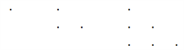1                      3                          6

Example: What is the 312th term of the series 1, 2, 2, 3, 3, 3, 4, 4, 4, 4, 5, 5, 5, 5, 5,…………..?

a) 24

b) 22

c) 25

d) 20

Solution:

You are required to define the pattern in the series in variables.

Here 1 is the first term, the next term is 2 till the 3rd term, 3 till the 6th term, 4 till the 10th term, n till the n(n+1)/2 th term.

Thus, 210th term will be = 20 as (20 × 21)/2 = 210.

So, 300th term = 24, because (24 × 25)/2 = 300.

Next 25 terms will be 25. So, the required answer is 25.

• #### Sum of consecutive triangular numbers will be square numbers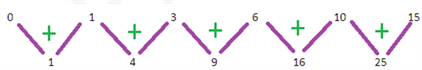What is a square number?? Quite simply, it is a “square”.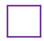Example: 1, 3, 6, 10, 15, 21,……….. is the series of numbers up to n.

This is logical because the sum of two triangles is a square.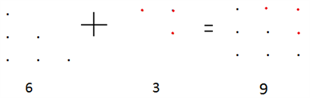9 is a perfect square. Now you graphically know why a square number is called a square.

• #### Difference between squares of triangular numbers will be cube numbers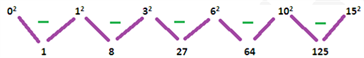Example: Sum of squares of first “n” natural numbers

12+22+32+42+……….n2

∑n²= (n(n+1)×(2n+1))/6

Important Application:

Find the total number of squares in a Chessboard is an 8 × 8 square.

Total number of squares in an n × n square = ∑n2

We can explain this method using the unitary method (i.e. by finding out the pattern using smaller values of n) In a 1×1 square,

Total no.of squares = 1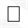In a 2 × 2 square there will be 4 squares of area 1 cm²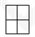And 1 square of area 4 cm² Total squares = 1 + 4 = 5.

In a 3 × 3 square there will be 9 squares of area 1cm²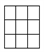4 squares of area 4 cm² and 1 square of area 9cm² Total = 1 + 4 + 9.

By following the pattern, total number of squares in an n × n square = ∑n2 = (n(n+1) (2n+1))/6.

∴ A Chessboard is an 8 × 8 square, by substituting n = 8 in the above equation we will get the total number of squares on a chess board as ((8×9×17))/6 = 204.

### Illustrations:

1. A person wrote first N consecutive numbers (natural) on a board and found their sum. Another person came and deleted the smallest number and found the sum of remaining numbers. The process continued until N remained on the board.

The average of all the sums is found to out to be 105. Find N?

a) 17

b) 20

c) 19

d) 23

Solution:

1 2 3 4 5……. n

2 3 4 5……. n

3 4 5……. n

4 5……. n

Sum of all terms of the series above = (1) +(2 + 2) +( 3 + 3 + 3)…….(n + n + n…n times)

= 12 + 22 + 32+……..n2

= ∑n2

Average of all sums = ∑ n2/n = (n(n+1) (2n+1))/6n = ((n+1) (2n+1))/6 = 105

=> (n+1)(2n+1) = 630 => n = 17.

2. Find the sum of the first 20 terms of the series 2 × 3, 4 × 6, 6 × 9, 8 × 12,………..

a) 10710

b) 12654

c) 14880

d) 17220

Solution:

In these kinds of question, the 1st step is to find out the nth term of the series by trial and error ( by following the pattern).

Here, nth term = 2n × 3n

Now take 2n × 3n = 6n2 = 6 × (n(n+1).(2n+1))/6 = n ( n+1 ) ( 2n+ 1)

Then put n = 20 to get the required answer as 17220.

• #### Sum of cubes of first “n” natural numbers

13 + 23 + 33 +……………n3

∑n3= (n2 (n+1)2)/4 = [n(n+1)/2]2 = (∑n)2

### Important Applications

#### 1. Total number of rectangles in an n × n square

= ∑n3= (n2 (n+1)2)/4

In an n × n square there will be (n + 1) horizontal lines and (n + 1) vertical lines.

To form a rectangle we need to select 2 horizontal lines from (n + 1) horizontal lines in (n+1)C2 ways and 2 vertical lines from(n + 1) vertical lines in (n+1)C2 ways.

So the number of rectangles =(n+1)C2x (n+1)C2= (n2 (n+1)2)/4 = (∑n)2 =∑n3

Example: Find the number of rectangles in a chess board A chess board is an 8 × 8 square.

Solution:

Here n=8.

Number of rectangles= ?n3= (n2 (n+1)2)/4 = (?n)2 = [(8 × 9)/2]2 = 1296.

#### 2.  Fractions in series

Evaluate 1/1×2+1/2×3+1/3×4…..+1/99×100

Each term can be written as 1/1×2= 1-1/2

1/2×3=1/2-1/3

.

.

1/99×100=1/99–1/100

Thus the series can be generalised as follows

1/n(n+1) = [1/n–1/(n+1)]

Look at this example,

Find the sum of the series N = 1/3 + 1/15 + 1/35 ………… 100 terms

a) 99/999

b) 1/199

c) 100/201

d) none of these

Solution:

1/3=1/3×1

1/15=1/3×5

1/35=1/((7×5) )…..

Here the difference d = 2,

Thus the sum can be written as ½x[1-1/3+1/3-1/5+1/5-1/7+1/7………………………………………+1/199-1/201] (1/2)[1-1/201]=200/2×201=100/201

It thus follows that

1/n(n+d) = [1/n–1/(n+d)] (in the case above, d=2)

Example: Evaluate (1 – 5/6 + 7/12 – 9/20 + 11/30 – 13/42 + 15/56 – 17/72 + 19/90)

Solution:

Each term can be expressed as the sum of two fractions as follows:

5/6 = 1/2 + 1/3

7/12 = 1/3 + 1/4 . .

19/90 = 1/9 + 1/10

This reduces to 1 – (1/2 + 1/3) + (1/3 + 1/4) – (1/4 + 1/5)……. + (1/9 + 1/10) = 1 – 1/2 + 1/10 = 3/5

Stay tuned with Byju’s to get more lessons on important CAT topics. Byju’s also provide the CAT aspirants with the latest CAT exam syllabus, pattern, sample papers and several video lessons to help them prepare more effectively and ace the CAT easily.